# JP2012060221A - Sequential comparison type analog-digital conversion circuit, and receiver - Google Patents

## Info

Publication number
JP2012060221A
JP2012060221A JP2010198812A JP2010198812A JP2012060221A JP 2012060221 A JP2012060221 A JP 2012060221A JP 2010198812 A JP2010198812 A JP 2010198812A JP 2010198812 A JP2010198812 A JP 2010198812A JP 2012060221 A JP2012060221 A JP 2012060221A
Authority
JP
Japan
Prior art keywords
capacitor
capacitance
set
parasitic
comparator
Prior art date
Legal status (The legal status is an assumption and is not a legal conclusion. Google has not performed a legal analysis and makes no representation as to the accuracy of the status listed.)
Granted
Application number
JP2010198812A
Other languages
Japanese (ja)
Other versions
JP5204176B2 (en
Inventor
Mai Araki
Masanori Furuta

Original Assignee
Toshiba Corp

Priority date (The priority date is an assumption and is not a legal conclusion. Google has not performed a legal analysis and makes no representation as to the accuracy of the date listed.)
Filing date
Publication date
Application filed by Toshiba Corp, 株式会社東芝 filed Critical Toshiba Corp
Priority to JP2010198812A priority Critical patent/JP5204176B2/en
Publication of JP2012060221A publication Critical patent/JP2012060221A/en
Application granted granted Critical
Publication of JP5204176B2 publication Critical patent/JP5204176B2/en
Application status is Expired - Fee Related legal-status Critical
Anticipated expiration legal-status Critical

## Images

•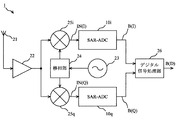•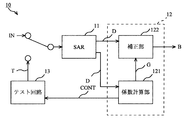•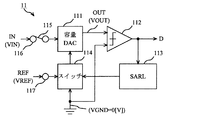•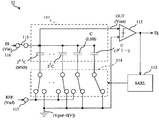•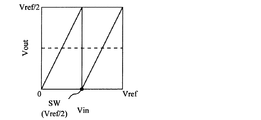•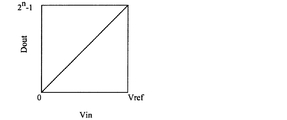••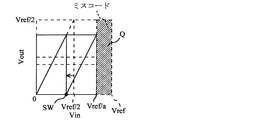•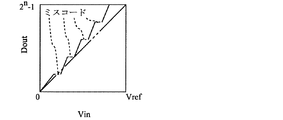•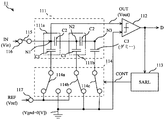•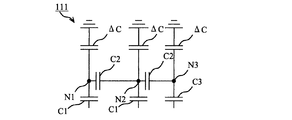•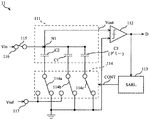•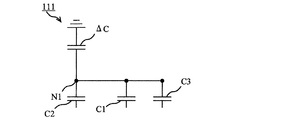•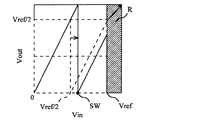•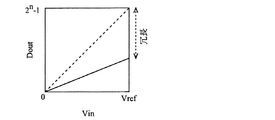•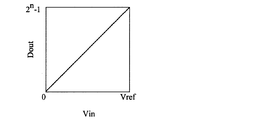•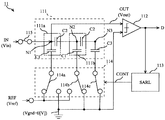## Classifications

• HELECTRICITY
• H03BASIC ELECTRONIC CIRCUITRY
• H03MCODING; DECODING; CODE CONVERSION IN GENERAL
• H03M1/00Analogue/digital conversion; Digital/analogue conversion
• H03M1/12Analogue/digital converters
• H03M1/34Analogue value compared with reference values
• H03M1/38Analogue value compared with reference values sequentially only, e.g. successive approximation type
• H03M1/46Analogue value compared with reference values sequentially only, e.g. successive approximation type with digital/analogue converter for supplying reference values to converter
• H03M1/466Analogue value compared with reference values sequentially only, e.g. successive approximation type with digital/analogue converter for supplying reference values to converter using switched capacitors
• HELECTRICITY
• H03BASIC ELECTRONIC CIRCUITRY
• H03MCODING; DECODING; CODE CONVERSION IN GENERAL
• H03M1/00Analogue/digital conversion; Digital/analogue conversion
• H03M1/66Digital/analogue converters
• H03M1/74Simultaneous conversion
• H03M1/78Simultaneous conversion using ladder network

## Abstract

PROBLEM TO BE SOLVED: To reduce a circuit area of a sequential comparison type analog-digital conversion circuit (SAR-DAC).SOLUTION: An SAR11 includes an input terminal 116, a reference terminal 117, a first capacitor set 111a, a second capacitor set 111b, a dummy capacitor, a comparator 112, a switch 114, and an SARL113. The first capacitor set 111a includes a first capacitor having a first capacitance, and a second capacitor having a second capacitance which is twice the sum of the first capacitance and a parasitic capacitance which is parasitic on the first capacitor set. The dummy capacitor has a third capacitance which is less than the second capacitance. The comparator 112 compares a ground voltage with an output voltage corresponding to the electric charges accumulated in the first capacitor and the second capacitor of the first capacitor set 111a, and outputs the comparison result. The SARL113 switches the switch 114 for controlling an output voltage based on the comparison result of the comparator 112.

## Description

Embodiments described herein relate generally to a successive approximation analog-digital conversion circuit (hereinafter referred to as “SAR-ADC (Successive Approximation Register-Analog Digital Converter)”) and a receiving apparatus.

In recent years, high-speed wireless communication devices, video equipment, and the like have a tendency to realize an analog-digital conversion circuit (hereinafter referred to as “ADC (Analog Digital Converter)”) having a sampling frequency of gigahertz on the silicon-on-chip basis. Accordingly, there is a need for ADCs with high resolution, low power consumption, and small area.

As such an ADC, SAR-ADC is known. The SAR-ADC is realized by a comparator, a capacitor digital-analog converter circuit (hereinafter referred to as “DAC (Digital Analog Converter)”), and a simple logic circuit. The SAR-ADC has attracted attention as a low power consumption and small area ADC.

In the SAR-ADC, when the weight of the capacitive DAC is an ideal binary, an ideal digital output code can be obtained for an analog input signal. However, in practice, since the weight varies depending on the parasitic capacitance, there is a possibility that a signal that is not AD converted (hereinafter referred to as “miscode”) may occur. Accordingly, the SAR-ADC requires a digital correction circuit for correcting a miscode.

A conventional digital correction circuit corrects a miscode by giving redundancy to an ADC using a redundant conversion algorithm. However, the digital correction circuit using the conventional redundant conversion algorithm is complicated. As a result, the circuit area of the SAR-DAC increases.

F. Kuttner., "A 1.2V 10b 20MSample / s Non-Binary Successive Approximation ADC in 0.13um CMOS," ISSCC Dig. Tech Papers, pp. 176-177, Feb., 2002. S.M. Chen and R.W. Brodersen, "A 6-bit 600-MS / s 5.3-mW Asynchronous ADΔCn 0.13-um CMOS," IEEE J. Solid-State ΔCrcuits, Vol. 41, Dec. 2006, pp. 2669-80.

The circuit area of the digital correction circuit and the test circuit used together with the SAR-ADC is reduced.

According to the present embodiment, the SAR-ADC includes an input terminal, a reference terminal, a first capacitor set, a second capacitor set, a dummy capacitor, a comparator, a switch, and a logic circuit. An input voltage is applied to the input terminal. A reference voltage is applied to the reference terminal. The first capacitor set includes a first capacitor having a first capacity, and a second capacitor having a second capacity that is twice the sum of the first capacity and the parasitic capacity parasitic on the first capacitor set. An input terminal is connected to a node between the first capacitor and the second capacitor. The second capacitor set includes a first capacitor and a second capacitor, and the second capacitor of the first capacitor set is connected to a node between the first capacitor and the second capacitor. The dummy capacitor has a third capacitance less than the second capacitance, and is connected between the second capacitor of the second capacitor set and the ground. The comparator compares the output voltage corresponding to the charges accumulated in the first capacitor and the second capacitor of the first capacitor set with the ground voltage, and outputs a comparison result. The switch is connected between the first capacitor and the reference terminal of the first capacitor set and the second capacitor set. The logic circuit switches the switch based on the comparison result of the comparator in order to control the output voltage.

Schematic which shows the structure of the receiver 1 which concerns on this embodiment. Schematic which shows the detailed structure of SAR-ADC10i and 10q of FIG. Schematic which shows the structure of SAR11 of FIG. A model for explaining the operating principle of SAR11. 5 is a graph showing transfer characteristics (relationship between an input voltage Vin and an output voltage Vout) of the MSB (j = n) of the capacitor DAC 111 of FIG. 5 is a graph showing an overall transfer characteristic (relationship between an input voltage Vin and a digital output Dout) of the capacitor DAC 111 in FIG. 4. FIG. 5 is a schematic diagram illustrating parasitic capacitance that is parasitic on the capacitor DAC 111 in FIG. 4. 7 is a graph showing transfer characteristics (relationship between input voltage Vin and output voltage Vout) of the MSB (j = n) of the capacitor DAC 111 of FIG. 7 is a graph showing an overall transfer characteristic (a relationship between an input voltage Vin and a digital output Dout) of the capacitor DAC 111 in FIG. 6. Schematic which shows the structure of SAR11 which concerns on 1st Embodiment. FIG. 9 is a schematic diagram illustrating parasitic capacitance that is parasitic on the capacitor DAC 111 in FIG. 8; Schematic which shows the structure of SAR11 which concerns on 2nd Embodiment. FIG. 11 is a schematic diagram illustrating parasitic capacitance that is parasitic on the capacitor DAC 111 of FIG. 10. 11 is a graph showing transfer characteristics (relationship between input voltage Vin and output voltage Vout) of the MSB (j = n) of the capacitor DAC 111 of FIG. The graph which shows the transfer characteristic of SAR11 before correction | amendment by the correction | amendment part 122 of FIG. The graph which shows the transfer characteristic of SAR11 after the correction | amendment by the correction | amendment part 122 of FIG. Schematic which shows the structure of SAR11 which concerns on the modification of 1st Embodiment.

The present embodiment will be described with reference to the drawings.

First, the configuration of the receiving apparatus according to this embodiment will be described. FIG. 1 is a schematic diagram illustrating a configuration of a receiving device 1 according to the present embodiment.

As shown in FIG. 1, the receiving apparatus 1 includes an antenna 21, a low noise amplifier (hereinafter referred to as LNA (Low Noise Amplifier)) 22, a local oscillator 23, a phase shift unit 24, and frequency conversion units 25i and 25q. SAR-ADCs 10i and 10q, and a digital signal processing unit 26.

The antenna 21 in FIG. 1 is a module that receives a radio signal transmitted from a base station (not shown) and inputs the received radio signal to the LNA 22.

The LNA 22 in FIG. 1 is a module that amplifies a radio signal input from the antenna 21 to a predetermined level and inputs the amplified radio signal to the frequency conversion units 25i and 25q.

The local oscillator 23 of FIG. 1 is a module that generates a local signal necessary for converting a radio signal into an analog baseband signal and inputs the generated local signal to the phase shift unit 24.

The phase shifter 24 in FIG. 1 is a module that distributes the local signal input from the local oscillator 23 into two. More specifically, the phase shifter 24 inputs one local signal to the frequency converter 25i, delays the phase of the other local signal by 90 degrees, and inputs the delayed local signal to the frequency converter 25q.

The SAR-ADCs 10i and 10q in FIG. 1 convert the analog input signal IN input from the frequency conversion units 25i and 25q into a digital baseband signal B, and the converted digital baseband signal B to the digital signal processing unit 26, respectively. This is the module to input. The digital baseband signal B (I) converted by the SAR-ADC 10i is a digital signal converted from the in-phase signal. The digital baseband signal B (Q) converted by the SAR-ADC 10q is a digital signal converted from a quadrature signal. Details of the SAR-ADCs 10i and 10q will be described later.

The digital signal processing unit 26 in FIG. 1 decodes the digital baseband signal B input from the SAR-ADCs 10i and 10q, and uses the decoded digital baseband signal B (D) as a cellular phone chip or a wireless LAN (Local This is a module that outputs to a wireless communication device (not shown) such as an area network chip.

Next, details of the SAR-ADCs 10i and 10q in FIG. 1 will be described. FIG. 2 is a schematic diagram showing a detailed configuration of the SAR-ADCs 10i and 10q in FIG. Since the configuration of the SAR-ADC 10i and the configuration of the SAR-ADC 10q in FIG. 1 are the same, the SAR-ADCs 10i and 10q are hereinafter referred to as SAR-ADC10. The analog input signals IN (I) and IN (Q) in FIG. 1 are referred to as analog input signals IN. The digital baseband signals B (I) and B (Q) in FIG.

As shown in FIG. 2, the SAR-ADC 10 includes a successive approximation register (hereinafter referred to as “SAR (Successive Approximation Register)”) 11, a digital correction circuit 12, and a test circuit 13.

The SAR 11 in FIG. 2 is an n (n is an integer of 2 or more) bit converter weighted with binary weights. The SAR 11 converts the analog input signal IN input from the frequency converters 25i and 25q in FIG. 1 or the analog test signal T input from the test circuit 13 into a digital output code D, and digitally corrects the converted digital output code D Input to the circuit 12. Details of the SAR 11 will be described later.

The digital correction circuit 12 in FIG. 2 is a circuit that corrects the digital output code D input from the SAR 11. The digital correction circuit 12 includes a coefficient calculation unit 121 and a correction unit 122.

The coefficient calculation unit 121 in FIG. 2 is a module that calculates a correction coefficient G used to correct the digital output code D. The digital output code D input from the SAR 11 to the coefficient calculation unit 121 corresponds to the analog test signal T. The coefficient calculation unit 121 calculates a correction coefficient G based on the digital output code D corresponding to the analog test signal T, and inputs the calculated correction coefficient G to the correction unit 122. The correction coefficient G is constant regardless of the number of bits of SAR11 (value of n).

The correction unit 122 in FIG. 2 generates the digital baseband signal B by correcting the digital output code D input from the SAR 11 using the correction coefficient G input from the coefficient calculation unit 121, and generates the generated digital base This is a module for inputting the band signal B to the digital signal processing unit 26 of FIG. The digital output code D input from the SAR 11 to the correction unit 122 corresponds to the analog input signal IN. Details of the correction unit 122 will be described later.

The test circuit 13 in FIG. 2 is a circuit that generates an analog test signal T based on the control signal CONT input from the coefficient calculation unit 121. For example, the test circuit 13 generates the analog test signal T using a reference voltage or a ground voltage described later. Thereby, the circuit scale of the test circuit 13 can be reduced.

Next, details of the SAR 11 in FIG. 2 will be described. FIG. 3 is a schematic diagram showing the configuration of the SAR 11 in FIG.

As illustrated in FIG. 3, the SAR 11 includes a capacitor DAC 111, a comparator 112, a successive approximation logic circuit (hereinafter referred to as “SARL (Successive Approximation Register Logic)”) 113, switches 114 and 115, and an input terminal 116. And a reference terminal 117.

An input signal IN is input to the input terminal 116 in FIG. That is, the voltage of the input signal IN (hereinafter referred to as “input voltage”) Vin is applied to the input terminal 116.

A reference signal REF is input to the reference terminal 117 in FIG. In other words, the voltage of the reference signal REF (hereinafter referred to as “reference voltage”) Vref is applied to the reference terminal 117.

The capacitor DAC 111 in FIG. 3 is connected to the input terminal 116 and the reference terminal 117 or the ground. A switch 114 is connected between the reference terminal 117 and the ground and the capacitor DAC 111. A switch 115 is connected between the capacitor DAC 111 and the input terminal 116. When the switches 114 and 115 are switched, the input voltage Vin and the ground voltage Vgnd (Vgnd = 0 [V]) are supplied to the capacitor DAC 111. The capacitor DAC 111 inputs an output signal OUT based on the input voltage Vin and the ground voltage Vgnd to the comparator 112.

3 is supplied with the voltage of the output signal OUT (hereinafter referred to as “output voltage Vout”) and the ground voltage Vgnd. The comparator 112 compares the output voltage Vout and the ground voltage Vgnd, and inputs the digital output code D based on the comparison result to the coefficient calculation unit 121 or the correction unit 122 and the SARL 113 in FIG. For example, when the output voltage Vout is equal to or higher than the ground voltage Vgnd, the digital output code D is “1” (high), and when the output voltage Vout is lower than the ground voltage Vgnd, the digital output code D is “0”. (Low).

The SARL 113 in FIG. 3 is a logic circuit that switches the switch 114 based on the digital output code D input from the comparator 112. As a result, the voltage (reference voltage Vref or ground voltage Vgnd) supplied to the capacitor DAC 111 changes.

In other words, in the SAR 11 of FIG. 3, the analog input signal IN is sampled by the capacitor DAC 111, and the voltage (reference voltage Vref or ground voltage Vgnd) supplied to the capacitor DAC 111 changes based on the comparison result of the comparator 112. Thereby, the digital output code D corresponding to the output signal OUT is obtained.

Next, the operation principle of SAR-ADC will be described. FIG. 4 is a model for explaining the operation principle of the SAR 11.

The SAR 11 in FIG. 4 includes an n-bit capacitor DAC 111 using a capacitor DAC weighted by binary weights, a comparator 112, a SARL 113, and a switch 114.

The capacitor DAC 111 in FIG. 4 includes n capacitors and dummy capacitors. When the number of bits corresponding to the capacitor is j (j is an integer of 1 to n), the capacitance of the capacitor corresponding to j bits is 2 j−1 C. That is, the capacitance of the capacitor corresponding to the least significant bit (hereinafter referred to as “LSB (Least Significant Bit)”) (j = 1) is 2 0 C (= C), and the most significant bit (hereinafter referred to as “MSB (Most The capacitance of the capacitor corresponding to (significant bit) ”(j = n) is 2 n−1 C. The capacity of the dummy capacitor is the same C as the capacitor corresponding to the LSB.

The SAR 11 in FIG. 4 operates in the order of sampling and AD conversion.

In sampling, charges corresponding to the potential difference between the input voltage Vin and the ground voltage Vgnd are accumulated in all capacitors of the capacitor DAC 111. Thereby, the input signal IN is sampled.

In AD conversion, the switch 114 is switched according to the comparison result of the comparator 112. Thereby, the reference terminal 117 or the ground is connected to n capacitors excluding the dummy capacitors. Specifically, when the digital output code Dj, which is a j-bit digital output code, is “1”, a capacitor corresponding to the j bit is connected to the reference terminal 117 and the digital output code Dj is “0”. In some cases, a capacitor corresponding to j bits is connected to ground. Thereby, the analog input signal IN is converted into the digital output code Dj.

Equation 1 represents the output voltage Vout in the n-bit successive approximation operation of the capacitor DAC 111 in FIG. As represented by Equation 1, in the n-bit successive approximation operation of the capacitor DAC 111, AD conversion is performed in binary. Accordingly, the radix (RADIX) of the capacitor DAC 111 is 2.

FIG. 5A is a graph showing the transfer characteristics (relationship between the input voltage Vin and the output voltage Vout) of the MSB (j = n) of the capacitor DAC 111 of FIG. FIG. 5B is a graph showing an overall transfer characteristic (relationship between the input voltage Vin and the digital output Dout) of the capacitor DAC 111 of FIG. The digital output Dout is a binary representation of the digital output code D expressed in decimal. The output code for each sample is represented by a straight line, with one sample per output code.

As shown in FIG. 5A, Vout = Vin when 0 ≦ Vin ≦ Vref / 2, and Vout = Vin−Vref / 2 when Vin> Vref / 2. That is, the switch 114 in FIG. 4 is switched at a point where Vin = Vref / 2 (hereinafter referred to as “switching point SW”). On the other hand, as shown in FIG. 5B, the output voltage Vout has a one-to-one correspondence with the input voltage Vin. That is, when the output voltage Vout is determined, the input voltage Vin is determined. The range of the digital output Dout (hereinafter referred to as “output range”) reaches full scale (2 n −1).

Next, the capacitor DAC 111 in FIG. 4 in consideration of parasitic capacitance will be described. FIG. 6 is a schematic diagram for explaining a parasitic capacitance parasitic to the capacitor DAC 111 of FIG.

As shown in FIG. 6, the parasitic capacitance of the capacitor ΔC is parasitic on the node on the input terminal 116 side. The output voltage Vout in the MSB (j = n) successive approximation operation is expressed by Equation 2.

FIG. 7A is a graph showing transfer characteristics (relationship between the input voltage Vin and the output voltage Vout) of the MSB (j = n) of the capacitor DAC 111 of FIG. FIG. 7B is a graph showing an overall transfer characteristic (relationship between the input voltage Vin and the digital output Dout) of the capacitor DAC 111 of FIG.

FIG. 7A shows that the range of the input voltage Vin (hereinafter referred to as “input range”) decreases due to the influence of the parasitic capacitance, as compared with FIG. 5A. In Equation 2, since ΔC is larger than 0, the coefficient of the reference voltage Vref is smaller than ½. That is, the cardinal number (RADIX) of SAR11 is larger than 2. Therefore, as shown in FIG. 7A, the switching point SW is shifted in the direction in which the input voltage Vin decreases. Thereby, the input voltage Vin of the switching point SW becomes smaller than Vref / 2. As a result, a miscode occurs in the region Q (Vref / a <Vin <Vref, 1 <a <2) outside the input range. On the other hand, as shown in FIG. 7B, the output voltage Vout does not correspond one-to-one with the input voltage Vin. That is, even if the output voltage Vout is determined, the input voltage Vin is not determined. In other words, FIG. 7B shows that a miscode occurs in each cycle due to a decrease in the input range after 2 bits (the broken line portion in FIG. 7B).

Next, details of the correction unit 122 and the SAR 11 in FIG. 2 will be described.

(First embodiment)
A first embodiment will be described. The first embodiment is an example in which the SAR 11 of FIG. 2 includes a SAR realized by a C-2C type capacitive DAC. In addition, the description about the content similar to the content already demonstrated is abbreviate | omitted.

FIG. 8 is a schematic diagram showing the configuration of the SAR 11 according to the first embodiment.

As illustrated in FIG. 8, the SAR 11 according to the first embodiment includes a capacitor DAC 111 realized by a C-2C capacitor DAC, a comparator 112, a SARL 113, switches 114 and 115, and an input terminal 116. A terminal 117. The comparator 112, the SARL 113, the switches 114 and 115, the input terminal 116, and the reference terminal 117 are the same as those in FIG.

The capacitor DAC 111 in FIG. 8 includes a first capacitor set 111a, a second capacitor set 111b, and a dummy capacitor having a third capacitor C3. Each of the first capacitor set 111a and the second capacitor set 111b includes a first capacitor having a first capacitor C1 and a second capacitor having a second capacitor C2 that is twice the first capacitor C1. The third capacitor C3 is the difference between the first capacitor C1 and a correction capacitor that is smaller than the first capacitor C1 and is equal to or greater than the parasitic capacitance that is parasitic on the first capacitor set 111a. The first capacitor of the first capacitor set 111a corresponds to the MSB. The first capacitor of the second capacitor set 111b corresponds to LSB. A switch 115 connected to the input terminal 116 and a comparator 112 are connected to a node N1 between the first capacitor and the second capacitor of the first capacitor set 111a. The second capacitor of the first capacitor set 111a is connected to the node N2 between the first capacitor and the second capacitor of the second capacitor set 111b. The node N3 to which the dummy capacitor is connected is connected to the second capacitor of the second capacitor set 111b. The dummy capacitor is connected to the ground via the switch 114c.

The first embodiment is an example of the capacitor DAC 111 realized by two capacitor sets (that is, a 2-bit C-2C type capacitor DAC), but n capacitor sets (that is, n-bit C-2C) are used. The present invention can also be applied to the capacitor DAC 111 realized by the mold capacitor DAC).

A parasitic capacitance parasitic to the capacitor DAC 111 in FIG. 8 will be described. FIG. 9 is a schematic diagram for explaining a parasitic capacitance parasitic to the capacitor DAC 111 of FIG.

As shown in FIG. 9, in the capacitor DAC 111 of FIG. 8, the parasitic capacitance of the capacitor ΔC is parasitic on each of the nodes N1 to N3. Hereinafter, description will be made on the assumption that the capacitances ΔC of the parasitic capacitances at the nodes N1 to N3 are equal.

The transfer characteristic when the switch 114a of FIG. 8 is connected to the reference terminal 117 and the switch 114b is connected to the ground is expressed by Equation 3.

The transfer characteristic when the switch 114a in FIG. 8 is connected to the ground and the switch 114b is connected to the reference terminal 117 is expressed by Expression 4.

Equations 3 and 4 indicate that the parasitic capacitance of the capacitor ΔC can be corrected by setting the first capacitor C1 that satisfies Equation 5 and the third capacitor C3 that satisfies Equation 6. ing.

By substituting Equation 5 and Equation 6 into Equation 3 and Equation 4, respectively, Equation 7 and Equation 8 are obtained.

From Equation 7 and Equation 8, Equation 9 is obtained. Equation 9 shows that even if the input range is reduced by half, setting the reference voltage Vref to twice can suppress the occurrence of miscode due to the decrease in the input range.

The first capacitor C1 and the second capacitor C2 are set so as to satisfy Expression 10, respectively. Thereby, the correction coefficient G becomes less than 2. As a result, as expressed in Expression 7, the MSB radix (RADIX) of the capacitor DAC 111 is less than 2. Further, as expressed in Equation 8, the radix other than MSB (RADIX) is 2.

FIG. 12 is a graph showing the transfer characteristics (relationship between the input voltage Vin and the output voltage Vout) of the MSB (j = n) of the capacitor DAC 111 of FIG. FIG. 13A is a graph showing transfer characteristics of the SAR 11 before correction by the correction unit 122 of FIG. FIG. 13B is a graph showing transfer characteristics of the SAR 11 after correction by the correction unit 122 of FIG.

As expressed in Equation 7, the coefficient of the reference voltage V′ref is greater than ½. That is, the radix of MSB (RADIX) is less than 2. Therefore, as shown in FIG. 12, the switching point SW shifts in the direction in which the input voltage Vin in FIG. 12 increases. FIG. 12 shows that the input range is expanded by eliminating the influence of the parasitic capacitance as compared with FIG. 5A. The amount R in which the input range is expanded corresponds to redundancy. In FIG. 13A, due to redundancy, the output range does not reach full scale (Vin = Vref, Dout = 2 n −1) that can be output in binary. In FIG. 13B, the output range has reached full scale.

In other words, the SAR 11 according to the first embodiment includes the input terminal 116, the reference terminal 117, the first capacitor set 111a, the second capacitor set 111b, the dummy capacitor, the comparator 112, the switch 114, the logic Circuit (SARL113). An input voltage Vin is applied to the input terminal 116. A reference voltage Vref is applied to the reference terminal 117. The first capacitor set 111a includes a first capacitor having a first capacitance C1, a second capacitor having a second capacitance C2 that is twice the sum of the first capacitance C1 and the parasitic capacitance parasitic on the first capacitor set, The input terminal 116 is connected to a node N1 between the first capacitor and the second capacitor. The second capacitor set 111b includes a first capacitor and a second capacitor, and the second capacitor of the first capacitor set 111a is connected to a node N2 between the first capacitor and the second capacitor. The dummy capacitor has a third capacitance C3 that is less than the second capacitance C2, and is connected between the second capacitor of the second capacitor set 111b and the ground. The comparator 112 compares the output voltage Vout corresponding to the charges accumulated in the first capacitor and the second capacitor of the first capacitor set 111a with the ground voltage Vgnd, and outputs a comparison result. The switch 114 is connected between the first capacitors of the first capacitor set 111a and the second capacitor set 111b and the reference terminal 117. The logic circuit (SARL 113) switches the switch 114 based on the comparison result of the comparator 112 in order to control the output voltage Vout.

Next, details of the correction unit 122 of FIG. 2 according to the first embodiment will be described.

As described above, the digital output code D having the transfer characteristics shown in FIG. 13A is input to the correction unit 122 shown in FIG.

The correction unit 122 in FIG. 2 uses a predetermined range correction coefficient depending on the correction coefficient G input from the coefficient calculation unit 121 to the digital output code D having the transfer characteristic in FIG. Multiply by H (H = G / 2). As a result, as shown in FIG. 13B, a digital output code D having the transfer characteristics of the SAR 11 whose output range reaches full scale (that is, the same transfer characteristics as in FIG. 5B) is obtained. The digital output code D having the transfer characteristics of FIG. 13B corresponds to the digital baseband signal B of FIG.

According to the first embodiment, the capacitance ΔC of the parasitic capacitance is set in advance using the parasitic capacitance extraction tool. Thereby, the correction coefficient G in FIG. 2 becomes constant, and the redundant conversion algorithm of the correction unit 122 is simplified. As a result, the circuit scales of the digital correction circuit 12 and the test circuit 13 can be reduced.

(Second Embodiment)
A second embodiment will be described. The second embodiment is an example in which the SAR 11 of FIG. 2 includes a SAR realized by a 2-bit binary weight type capacitor DAC. In addition, the description about the content similar to the content already demonstrated is abbreviate | omitted.

FIG. 10 is a schematic diagram illustrating the configuration of the SAR 11 according to the second embodiment.

As shown in FIG. 10, the SAR 11 according to the second embodiment includes a capacitor DAC 111 realized by a 2-bit binary weight capacitor DAC, a comparator 112, a SARL 113, switches 114 and 115, an input terminal 116, A reference terminal 117. The comparator 112, the SARL 113, the switches 114 and 115, the input terminal 116, and the reference terminal 117 are the same as those in FIG.

The capacitor DAC 111 in FIG. 10 includes a first capacitor having a first capacitor C1, a second capacitor having a second capacitor C2, and a dummy capacitor having a third capacitor C3. The first capacitor, the second capacitor, and the dummy capacitor are each connected in parallel. The second capacitor corresponds to the MSB. The first capacitor corresponds to LSB. A switch 115 and a comparator 112 are connected to the node N1. The dummy capacitor is connected to the input terminal 116 and the ground. The second capacitor C2 in FIG. 10 is set to satisfy Equation 11.

A parasitic capacitance parasitic to the capacitor DAC 111 in FIG. 10 will be described. FIG. 11 is a schematic diagram for explaining a parasitic capacitance parasitic to the capacitor DAC 111 of FIG.

As shown in FIG. 11, in the capacitor DAC 111 of FIG. 10, a parasitic capacitance of the capacitor ΔC is parasitic at the node N1.

The third capacitor C3 in FIG. 10 is set to satisfy Equation 12. In Equation 12, Ccal is a correction capacitance for correcting performance degradation due to parasitic capacitance. The correction capacitor Ccal is greater than or equal to the parasitic capacitance ΔC and less than the first capacitor C1.

In consideration of Expression 11 and Expression 12, the output voltage Vout is expressed by Expression 13. As expressed in Equation 13, the radix (RADIX) when converting the MSB of the capacitor DAC 111 is less than 2, and the coefficient of the reference voltage Vref is greater than ½.

FIG. 12 is a graph showing the transfer characteristics (relationship between the input voltage Vin and the output voltage Vout) of the MSB (j = n) of the capacitor DAC 111 of FIG.

As expressed in Equation 13, the coefficient of the reference voltage Vref is greater than ½. That is, the radix (RADIX) of the capacitor DAC 111 in FIG. Therefore, as shown in FIG. 12, the switching point SW shifts in the direction in which the input voltage Vin in FIG. 12 increases. FIG. 12 shows that the input range is expanded by eliminating the influence of the parasitic capacitance as compared with FIG. 5A. The amount R in which the input range is expanded corresponds to redundancy.

In other words, the SAR 11 according to the second embodiment includes an input terminal 116, a reference terminal 117, a first capacitor, a second capacitor, a dummy capacitor, a comparator 112, a switch 114, and a logic circuit (SARL 113). And comprising. An input voltage Vin is applied to the input terminal 116. A reference voltage Vref is applied to the reference terminal 117. The first capacitor has a first capacitor C1. The second capacitor has a second capacitance C2 that is twice the first capacitance C1. The dummy capacitor has a third capacitor C3. The third capacitor C3 is a difference between the first capacitor C1 and the correction capacitor Ccal. The correction capacitance Ccal is smaller than the first capacitance C1 of the first capacitor and is equal to or greater than the capacitance ΔC of the parasitic capacitance parasitic to the first capacitor. The comparator 112 compares the output voltage Vout corresponding to the charges accumulated in the first capacitor, the second capacitor, and the dummy capacitor with the ground voltage Vgnd, and outputs a comparison result. The switch 114 is connected between the first capacitor and the second capacitor and the reference terminal 117. The logic circuit (SARL 113) controls the switch 114 based on the comparison result of the comparator 112 in order to control the output voltage Vout.

The correction unit 122 and the coefficient calculation unit 121 in FIG. 2 according to the second embodiment are the same as those in the first embodiment.

According to the second embodiment, the parasitic capacitance capacitance ΔC is estimated in advance using the parasitic capacitance extraction tool, and the third capacitance C3 of the dummy capacitor that satisfies Equation 12 is set within a range in which redundancy is ensured. Thereby, the correction coefficient G in FIG. 2 becomes constant, and the redundant conversion algorithm of the correction unit 122 is simplified. As a result, the circuit scales of the digital correction circuit 12 and the test circuit 13 can be reduced.

The second embodiment is an example in which the third capacitor C3 of the dummy capacitor that satisfies Equation 12 is set in the capacitor DAC 111 of FIG. 10 realized by the 2-bit binary weight capacitor DAC. In the capacitor DAC 111 of FIG. 8 realized by the DAC, the present invention can also be applied to an example in which the third capacitor C3 of the dummy capacitor satisfying Expression 12 is set (see FIG. 14).

Further, as the correction capacity Ccal is larger, more redundancy can be ensured. Thereby, the amount of mismatch error can be reduced. However, when the correction capacitance Ccal is large, the output range becomes small. Therefore, it is preferable to set an appropriate correction capacity Ccal according to the resolution required for the SAR 11.

In addition, this invention is not limited to embodiment mentioned above, It deform | transforms and implements a component in the range which does not deviate from the summary. Various inventions can be formed by appropriately combining a plurality of constituent elements disclosed in the above-described embodiments. For example, you may delete a some component from all the components shown by embodiment mentioned above. Furthermore, constituent elements over different embodiments may be appropriately combined.

11 SAR
111 capacity DAC
111a First capacitor set 111b Second capacitor set 112 Comparator 113 SARL
114, 115 switch 116 input terminal 117 reference terminal 12 digital correction circuit 121 coefficient calculation unit 122 correction unit 13 test circuit 21 antenna 22 LNA
23 Local Oscillator 24 Phase Shifter 25 Frequency Converter 26 Digital Signal Processor

## Claims (8)

1. An input terminal to which an input voltage is applied;
A reference terminal to which a reference voltage is applied;
A first capacitor having a first capacitance; and a second capacitor having a second capacitance that is twice the sum of the first capacitance and the parasitic capacitance parasitic on the first capacitor set; and A first capacitor set having the input terminal connected to a node between the second capacitor and the second capacitor;
A second capacitor set comprising: the first capacitor; and the second capacitor, wherein a second capacitor of the first capacitor set is connected to a node between the first capacitor and the second capacitor;
A dummy capacitor having a third capacitance less than the second capacitance and connected between a second capacitor of the second capacitor set and a ground;
A comparator that compares an output voltage corresponding to charges accumulated in the first capacitor and the second capacitor of the first capacitor set with a ground voltage, and outputs a comparison result;
A switch connected between the first capacitor of the first capacitor set and the second capacitor set and the reference terminal;
A logic circuit for switching the switch based on a comparison result of the comparator to control the output voltage;
A successive approximation type analog-digital conversion circuit characterized by comprising:
2.   2. The successive approximation analog according to claim 1, wherein the third capacitor is a difference between the first capacitor and a correction capacitor that is smaller than the first capacitor and is equal to or more than a parasitic capacitor that is parasitic on the first capacitor set. Digital conversion circuit.
3. An input terminal to which an input voltage is applied;
A reference terminal to which a reference voltage is applied;
A first capacitor having a first capacitance;
A second capacitor having a second capacitance twice as large as the first capacitance;
A dummy capacitor having a third capacitance that is a difference between the first capacitance and a correction capacitance that is smaller than the first capacitance and is equal to or greater than a parasitic capacitance parasitic to the first capacitor;
A comparator that compares an output voltage corresponding to charges accumulated in the first capacitor, the second capacitor, and the dummy capacitor with a ground voltage, and outputs a comparison result;
A switch connected between the first capacitor and the second capacitor and the reference terminal;
A logic circuit for controlling the switch based on a comparison result of the comparator to control the output voltage;
A successive approximation type analog-digital conversion circuit characterized by comprising:
4. An input terminal to which an input voltage is applied;
A reference terminal to which a reference voltage is applied;
A first capacitor having a first capacitance; and a second capacitor having a second capacitance that is twice the sum of the first capacitance and the parasitic capacitance parasitic on the first capacitor set; and A first capacitor set having the input terminal connected to a node between the second capacitor and the second capacitor;
A second capacitor set comprising: the first capacitor; and the second capacitor, wherein a second capacitor of the first capacitor set is connected to a node between the first capacitor and the second capacitor;
A dummy capacitor having a third capacitance less than the second capacitance and connected between a second capacitor of the second capacitor set and a ground;
A comparator that compares an output voltage corresponding to charges accumulated in the first capacitor and the second capacitor of the first capacitor set with a ground voltage, and outputs a comparison result;
A switch connected between the first capacitor of the first capacitor set and the second capacitor set and the reference terminal;
A logic circuit that switches the switch based on a comparison result of the comparator to control the output voltage; and a successive approximation type analog-digital conversion circuit,
A digital correction circuit for correcting the comparison result output by the comparator;
A receiving apparatus comprising:
5.   5. The receiving device according to claim 4, wherein the third capacitor is a difference between the first capacitor and a correction capacitor that is smaller than the first capacitor and is equal to or more than a parasitic capacitor parasitic on the first capacitor set.
6.   The receiving device according to claim 4, further comprising a test circuit that generates an analog test signal to be supplied to the digital correction circuit using the reference terminal or the ground terminal.
7. An input terminal to which an input voltage is applied;
A reference terminal to which a reference voltage is applied;
A first capacitor having a first capacitance;
A second capacitor having a second capacitance twice as large as the first capacitance;
A dummy capacitor having a third capacitance that is a difference between the first capacitance and a correction capacitance that is smaller than the first capacitance and is equal to or greater than a parasitic capacitance parasitic to the first capacitor;
A comparator that compares an output voltage corresponding to charges accumulated in the first capacitor, the second capacitor, and the dummy capacitor with a ground voltage, and outputs a comparison result;
A switch connected between the first capacitor and the second capacitor and the reference terminal;
A logic circuit that controls the switch based on a comparison result of the comparator to control the output voltage; and a successive approximation type analog-digital conversion circuit,
A digital correction circuit for correcting the comparison result output by the comparator;
A receiving apparatus comprising:
8.   The receiving apparatus according to claim 7, further comprising a test circuit that generates an analog test signal to be supplied to the digital correction circuit using the reference terminal or the ground terminal.
JP2010198812A 2010-09-06 2010-09-06 Successive comparison type analog-digital conversion circuit and receiver Expired - Fee Related JP5204176B2 (en)

## Priority Applications (1)

Application Number Priority Date Filing Date Title
JP2010198812A JP5204176B2 (en) 2010-09-06 2010-09-06 Successive comparison type analog-digital conversion circuit and receiver

## Applications Claiming Priority (2)

Application Number Priority Date Filing Date Title
JP2010198812A JP5204176B2 (en) 2010-09-06 2010-09-06 Successive comparison type analog-digital conversion circuit and receiver
US13/035,202 US8416115B2 (en) 2010-09-06 2011-02-25 Successive approximation register-analog digital converter and receiver

## Publications (2)

Publication Number Publication Date
JP2012060221A true JP2012060221A (en) 2012-03-22
JP5204176B2 JP5204176B2 (en) 2013-06-05

# Family

## Family Applications (1)

Application Number Title Priority Date Filing Date
JP2010198812A Expired - Fee Related JP5204176B2 (en) 2010-09-06 2010-09-06 Successive comparison type analog-digital conversion circuit and receiver

## Country Status (2)

US (1) US8416115B2 (en)
JP (1) JP5204176B2 (en)

## Cited By (2)

* Cited by examiner, † Cited by third party
Publication number Priority date Publication date Assignee Title
WO2015181682A1 (en) * 2014-05-28 2015-12-03 株式会社半導体エネルギー研究所 Analog-digital conversion circuit, semiconductor device, and electronic apparatus
WO2019224900A1 (en) * 2018-05-22 2019-11-28 オリンパス株式会社 Digital-to-analog converter, analog-to-digital converter, signal processing device, solid-state imaging device, and driving method

## Families Citing this family (11)

* Cited by examiner, † Cited by third party
Publication number Priority date Publication date Assignee Title
JP2012119767A (en) * 2010-11-29 2012-06-21 Toshiba Corp Successive approximation type a/d converter and dc-dc converter
JP2014120615A (en) * 2012-12-17 2014-06-30 Fujitsu Semiconductor Ltd Capacity element, capacity array, and a/d converter
US9316695B2 (en) * 2012-12-28 2016-04-19 Semiconductor Energy Laboratory Co., Ltd. Semiconductor device
US9300316B2 (en) 2014-02-28 2016-03-29 Qualcomm Incorporated Voltage doubling circuit for an analog to digital converter (ADC)
US9148159B1 (en) * 2014-03-13 2015-09-29 Texas Instruments Incorporated Dual comparator-based error correction scheme for analog-to-digital converters
US9337860B1 (en) * 2014-07-03 2016-05-10 Maxim Integrated Products, Inc. Precision sub-RADIX2 DAC with analog weight based calibration
CN104734718A (en) * 2015-02-03 2015-06-24 国网重庆市电力公司电力科学研究院 Hybrid DAC capacitor array structure
US9425814B1 (en) 2015-12-10 2016-08-23 Samsung Electronics Co., Ltd Redundancy scheme for flash assisted successive approximation register (SAR) analog-to-digital converter (ADC)
US9774345B1 (en) 2016-09-20 2017-09-26 Kabushiki Kaisha Toshiba Successive approximation register analog-to-digital converter
US9973202B2 (en) 2016-09-20 2018-05-15 Kabushiki Kaisha Toshiba Successive approximation register analog-to-digital converter
US9998162B2 (en) * 2016-09-30 2018-06-12 Intel Corporation Scalable stochastic successive approximation register analog-to-digital converter

## Citations (3)

* Cited by examiner, † Cited by third party
Publication number Priority date Publication date Assignee Title
JP2002314419A (en) * 2001-04-18 2002-10-25 Fuji Electric Co Ltd Analog/digital conversion circuit
JP2009118488A (en) * 2007-11-08 2009-05-28 Advantest Corp Da conversion device and ad conversion device
JP2010045723A (en) * 2008-08-18 2010-02-25 Fujitsu Ltd Digital-to-analog converter

## Family Cites Families (3)

* Cited by examiner, † Cited by third party
Publication number Priority date Publication date Assignee Title
US4097753A (en) * 1976-04-02 1978-06-27 International Business Machines Corporation Comparator circuit for a C-2C A/D and D/A converter
US7755521B1 (en) * 2008-12-23 2010-07-13 Advantest Corporation A-D convert apparatus, D-A convert apparatus and adjustment method
JP2011103576A (en) * 2009-11-11 2011-05-26 Renesas Electronics Corp Analog-to-digital converter

## Patent Citations (3)

* Cited by examiner, † Cited by third party
Publication number Priority date Publication date Assignee Title
JP2002314419A (en) * 2001-04-18 2002-10-25 Fuji Electric Co Ltd Analog/digital conversion circuit
JP2009118488A (en) * 2007-11-08 2009-05-28 Advantest Corp Da conversion device and ad conversion device
JP2010045723A (en) * 2008-08-18 2010-02-25 Fujitsu Ltd Digital-to-analog converter

## Cited By (2)

* Cited by examiner, † Cited by third party
Publication number Priority date Publication date Assignee Title
WO2015181682A1 (en) * 2014-05-28 2015-12-03 株式会社半導体エネルギー研究所 Analog-digital conversion circuit, semiconductor device, and electronic apparatus
WO2019224900A1 (en) * 2018-05-22 2019-11-28 オリンパス株式会社 Digital-to-analog converter, analog-to-digital converter, signal processing device, solid-state imaging device, and driving method

## Also Published As

Publication number Publication date
JP5204176B2 (en) 2013-06-05
US20120056770A1 (en) 2012-03-08
US8416115B2 (en) 2013-04-09

## Similar Documents

Publication Publication Date Title
Sahoo et al. A 12-bit 200-mhz cmos adc
US9100034B2 (en) Semiconductor integrated circuit device
US6448911B1 (en) Circuits and methods for linearizing capacitor calibration and systems using the same
Liu et al. A 1V 11fJ/conversion-step 10bit 10MS/s asynchronous SAR ADC in 0.18 µm CMOS
US6894627B2 (en) Increasing the SNR of successive approximation type ADCs without compromising throughput performance substantially
US7161512B1 (en) Gain error correction in an analog-to-digital converter
US7884749B2 (en) A/D converting apparatus
US8350740B2 (en) A/D conversion circuit and receiver
Ingino et al. A continuously calibrated 12-b, 10-MS/s, 3.3-VA/D converter
US7242338B2 (en) Differential digital-to-analog converter
US20160134300A1 (en) Sar adc and method thereof
USRE42878E1 (en) Analog-to-digital converting system
US7286074B2 (en) Semiconductor integrated circuit for communication including analog-to-digital conversion circuit
EP1182781B1 (en) Multistage converter employing digital dither
US7187318B1 (en) Pipeline ADC using multiplying DAC and analog delay circuits
US7501965B2 (en) Correcting for errors that cause generated digital codes to deviate from expected values in an ADC
US7397409B2 (en) Multi-bit pipeline analog-to-digital converter having shared amplifier structure
US6538594B1 (en) Methods and circuits for compensating for finite common mode rejection in switched capacitor circuits
Lin et al. A 9-bit 150-MS/s 1.53-mW subranged SAR ADC in 90-nm CMOS
Jo et al. 26.4 A 21fJ/conv-step 9 ENOB 1.6 GS/S 2× time-interleaved FATI SAR ADC with background offset and timing-skew calibration in 45nm CMOS
Alpman et al. A 1.1 v 50mw 2.5 gs/s 7b time-interleaved c-2c sar adc in 45nm lp digital cmos
Hong et al. A 7b 1GS/s 7.2 mW nonbinary 2b/cycle SAR ADC with register-to-DAC direct control
US9654132B2 (en) Hybrid charge-sharing charge-redistribution DAC for successive approximation analog-to-digital converters
US7030799B2 (en) Current-steering digital-to-analog converter
Chen et al. A 9b 100ms/s 1.46 mw sar adc in 65nm cmos

## Legal Events

Date Code Title Description
A621 Written request for application examination

Free format text: JAPANESE INTERMEDIATE CODE: A621

Effective date: 20120809

A977 Report on retrieval

Free format text: JAPANESE INTERMEDIATE CODE: A971007

Effective date: 20121210

A131 Notification of reasons for refusal

Free format text: JAPANESE INTERMEDIATE CODE: A131

Effective date: 20121214

A521 Written amendment

Free format text: JAPANESE INTERMEDIATE CODE: A523

Effective date: 20121226

TRDD Decision of grant or rejection written
A01 Written decision to grant a patent or to grant a registration (utility model)

Free format text: JAPANESE INTERMEDIATE CODE: A01

Effective date: 20130122

A61 First payment of annual fees (during grant procedure)

Free format text: JAPANESE INTERMEDIATE CODE: A61

Effective date: 20130214

FPAY Renewal fee payment (event date is renewal date of database)

Free format text: PAYMENT UNTIL: 20160222

Year of fee payment: 3

LAPS Cancellation because of no payment of annual fees